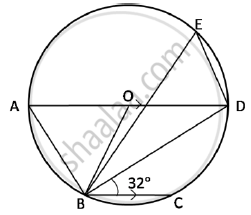Share

# In the Figure Given Below, an Ad is a Diameter. O is the Centre of the Circle Ad is Parallel to Bc and Angle Cbd = 32°. Find - ICSE Class 10 - Mathematics

ConceptCircumscribing and Inscribing a Circle on a Triangle

#### Question

In the figure given below, an AD is a diameter. O is the centre of the circle

AD is parallel to BC and angle CBD = 32°. Find

1) angle OBD

2) angle AOB

3) angle BED#### Solution

1) AD is parallel to BC, i.e., OD is parallel to BC and BD is transversal

=> angle ODB = angle CBD = 32^@ .... (Alternate angles)

In ΔOBD

OD = OB ....(Radii of the same circle)

=> angle ODB = angle OBD = 32^@

2) AD is parallel to BC, i.e., AO is parallel to BC and OB is transversal

=> angle AOB = angle OBC  .... (Alternate angles)

=> angle OBC = angle OBD + angle DBC

=> angle OBC = 32^@ + 32^@

=> angle OBC = 64^@

=> angle AOB = 64^@

3) In ΔOAB,

OA = OB . . . .(Radii of the same circle)

=> angle OAB = angleOBA = x(say)

angle OAB + angle OBA + angle AOB = 180^@

=> x + x+ 64^@ = 180^@

=> 2x = 180^@ -64^@

=> 2x = 116^@

=> x = 58^@

=> angle OAB = 58^@

i.e angle DAB = 58^@

=> angle DAB = angle BED = 58^@  ...(Angles inscribed in the same arc are equal)

Is there an error in this question or solution?

#### APPEARS IN

2015-2016 (March) (with solutions)
Question 3.2 | 4.00 marks

#### Video TutorialsVIEW ALL 

Solution In the Figure Given Below, an Ad is a Diameter. O is the Centre of the Circle Ad is Parallel to Bc and Angle Cbd = 32°. Find Concept: Circumscribing and Inscribing a Circle on a Triangle.
S